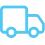Solved

# Variable UOM Conversion factors

• 2 replies
• 69 views

• Freshman I
• 8 replies

How does one deal with 2 uom’s where the conversion is not constant?

Example Steel industry

A pallet of sheets never weights the same. 100 sheets might weigh a ton with one PO Receipts and then next week 1.01 tons.

Also, what would be a solution where I Order in kg’s, I sell in Kg’s but I stock count in Sheets/each. what would be a good option for the base UOM? Remember I have variable UOM Conversion factor.

icon

Best answer by rosenjon 3 July 2022, 09:09

View original

### 2 replies

Userlevel 5+1

@apretorius62 We are in the metal industry. The way this is usually dealt with is via a conversion to tab weight. The calculation of tab weight for an item is usually done based on an “idealized” weight metric.

For example, you would take a standard density of material: https://www.sandmeyersteel.com/images/Alloy304-304L-APR2013.pdf

According to the above link, the density of T304 Stainless Steel is .285lb / cubic inch.

So if you have a 1” thick piece of T304 Stainless Steel, 20” X 20”, then we have 20 * 20 * 1 = 400 cubic inches.

400 in^3 * .285lb/in^3 = 114lbs

So, let’s say that we buy a pallet of 100 T304 sheets, 20 X 20. We pay \$10,000 for this pallet (random cost I made up).

Then to figure out our cost at tab, we multiply 114 lbs (weight of 1 plate at tab) * 100 (number of sheets we bought) = 11,400lbs of plate. Our cost at tab is \$10,000/11400lbs = \$0.877/lb

This allows you to have a standard conversion in your erp between say the area of a sheet of plate and its weight.

You don’t want a variable conversion factor for the sheet, trust me. The way I have described above is the correct way to handle this. It redistributes your cost and your selling price across a standard weight metric for the product. Then you can have standard conversions for UOM using the native Acumatica functionality (i.e. for a given size sheet, 1 sheet = 114 lbs = 400 sq in = etc).

Userlevel 5+1

Also, what would be a solution where I Order in kg’s, I sell in Kg’s but I stock count in Sheets/each. what would be a good option for the base UOM? Remember I have variable UOM Conversion factor.

Per above, you no longer have variable UOM Conversion factors. Acumatica recommends that you use the lowest common factor for the base UOM, so that you are always multiplying by some positive number to get other UOMs (this helps minimize rounding issues due to division). So the simplest answer is to try to figure out what the smallest common factor will be for you various UOMs (i.e. in most cases this well be the weight UOM, but in some cases it could be the EACH uom if the product weights less than a pound, for example). This can be determined on a per product basis.